Courses

# Test: Structural Isomerism- 2

## 18 Questions MCQ Test Chemistry for JEE | Test: Structural Isomerism- 2

Description
This mock test of Test: Structural Isomerism- 2 for JEE helps you for every JEE entrance exam. This contains 18 Multiple Choice Questions for JEE Test: Structural Isomerism- 2 (mcq) to study with solutions a complete question bank. The solved questions answers in this Test: Structural Isomerism- 2 quiz give you a good mix of easy questions and tough questions. JEE students definitely take this Test: Structural Isomerism- 2 exercise for a better result in the exam. You can find other Test: Structural Isomerism- 2 extra questions, long questions & short questions for JEE on EduRev as well by searching above.
QUESTION: 1

### Direction (Q. Nos. 1-8) This section contains 8 multiple choice questions. Each question has four  choices (a), (b), (c) and (d), out of which ONLY ONE option is correct.   Q. how many structural isomers (aldehyde + ketone) are possible for C5H10O ?

Solution: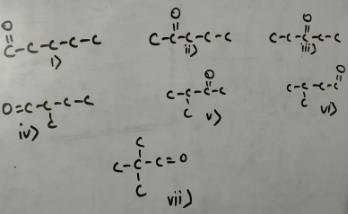We can see that for alkane, we atleast need alkane of 7 carbon atoms or C7H16.

QUESTION: 2

### An organic acid containing carbon, oxygen and hydrogen only has molar mass of 132 g. 6.6 g of this acid require 80 mL 1.25 M NaOH for complete neutralisation. How many carboxylic groups are present in one molecule of this acid?

Solution:

Let us consider organic acid as ‘A’.
Moles of A = mass of A/Molar mass = 6.6/132 = 0.05
Molarity of NaOH = 1.25M
And volume of NaOH  = 80 mL
Therefore, moles of NaOH = molarity × Volume
= 1.25×80×10-3 = 0.1 moles.
SO, 0.05 moles of A requires 0.1 moles of NaOH
1 mole of acid requires x moles of NaOH
x = 0.1/0.05×1 = 2
So for neutralisation of 1 mole of A, we need 2 moles of NaOH. Therefore given acid is dibasic and contains 2 -COOH groups.

QUESTION: 3

### An organic compound containing carbon, hydrogen and oxygen has molar mass 100. How many structural isomers satisfy this condition which are also simultaneously ketones?

Solution:

General formula
CnH2nO = 100
12×n+2×2n+16 = 100
n = 6
So, the formula of ketone is C6H12O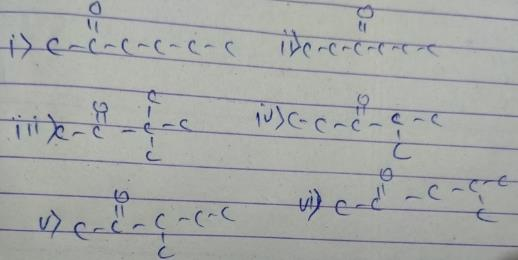So, according to me, the correct answer is 6 and not 8.

QUESTION: 4

Only two isomers of monochloro product is possible of

Solution: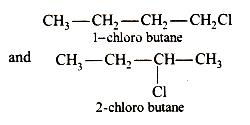QUESTION: 5

Which of the following compounds will exhibit cis-trans isomerism?

Solution:

The correct answer is Option A.
2-butene can show geometrical isomerism, due to different atoms/group of atoms attached on both sides of double bonded carbons as H and CH3 − groups.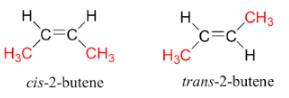QUESTION: 6

An organic compound has three ether isomers and it is the smallest ether which satisfy this condition. Which of the following is true regarding this compound?

Solution:

We know that for ether, we need C-O-C connectivity. Now, we can see that this arrangement won't give any isomer.So, we will increase one carbon atom.
We will get C-C-O-C. This also doesn’t give any isomer. SO we will add another carbon atom. This time, we will have C-C-C-O-C. With this arrangement, we can have 3 different isomers. They are :-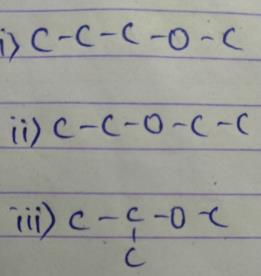So, we havegeneral fomula as C4H9OH. The different constitutional isomers of this lcohol are as follow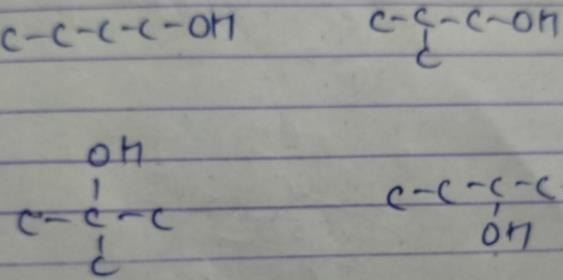We can see that there are 4 isomers of ths compound So option b is correct.

QUESTION: 7

How many structural isomers exist for C7H80 where each isomer contain a phenyl ring?

Solution:

First, we will check the Double bond equivalent. In C7H8O, DBE = 4,this means that we will have 3 double bonds and a ring and no additional double bond outside the ring.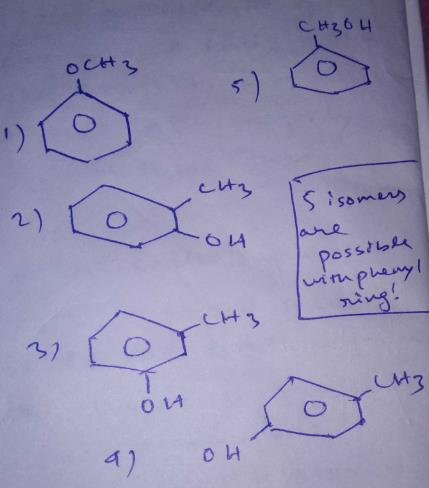These are the structural isomers that exists for C7H8O

QUESTION: 8

How many amide isomers are possible for C4H9ON ?

Solution: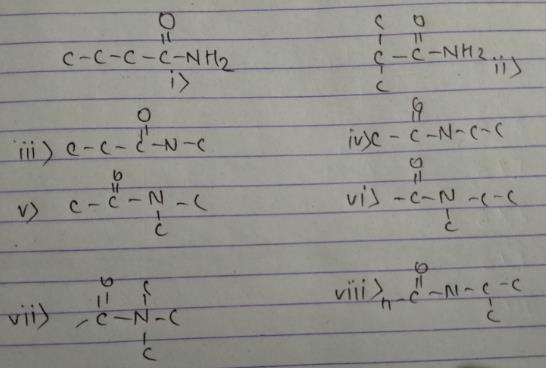These are 8 isomers of C4H9ON.

*Multiple options can be correct
QUESTION: 9

Direction (Q. Nos. 9-12) This section contains 4 multiple choice questions. Each question has four
choices (a), (b), (c) and (d), out of which ONE or  MORE THANT ONE  is correct.

Q.

Organic compound with molecular formula C2H4O2 can have which of the functional isomer(s)?

Solution:

The correct answer is option A,B
Functional groups having two oxygen atoms are " Easter " and " Carboxylic Acids " .
Compounds having molecular formula C2H4O2 have possible functional isomers. One is ethanoic acids or acetic acids ( Carboxylic Acids ) and other is METHYL Methanoate ( Ester ) ...
Acetic acids are colourless liquid organic compounds with the chemical formula CH3COOH ( also written as CH3CO2H or C2H4O2 ) .

*Multiple options can be correct
QUESTION: 10

The correct statement(s) about structural isomers of compounds having chemical composition C5H12O is/are

Solution: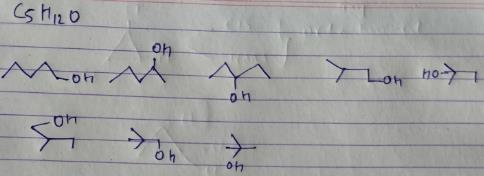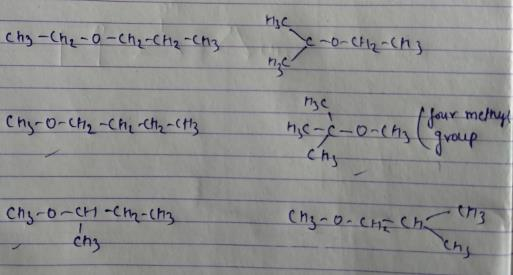All the 8 isomers of alcohol are intermolecular H-bonding.
There are 6 ethers which have H-bond acceptor(i.e O atm) but not H-bond donor(i.e H atom)
Also, the isomer with 4 methyl groups is mentioned above.
According to me, CH3CH2COCH2CH3 is metamer of CH3COCH2CH2CH3 OR CH3COCH(CH3)2
So all options are correct.

*Multiple options can be correct
QUESTION: 11

What is/are correct deduction regarding compounds with their composition C3H60?

Solution:
*Multiple options can be correct
QUESTION: 12

Which of the following on adding one molecule of hydrogen (H2) per molecule of the compound can result in compound capable of exhibiting stereoisomers?

Solution: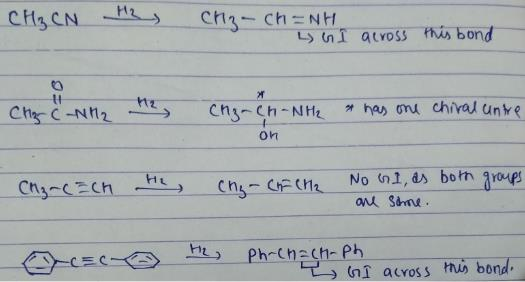So, option a, b and d are correct.

QUESTION: 13

Direction (Q. Nos. 13 and 14) Choice the correct combination of elements and column I and coloumn II  are given as option (a), (b), (c) and (d), out of which ONE option is correct.

Q.

Column I has some molecular formula and Column II has some organic functional groups. Match the functional group from Column II with the formula from Column I that may contain this functional group. Additional functional groups may also be present. There may also be more than one same functional groups present.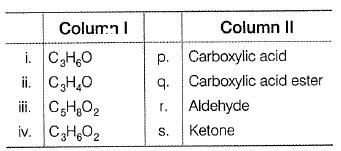Codes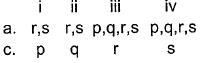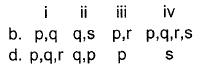Solution:
QUESTION: 14

Match the formula from column I with their possible identity from Column II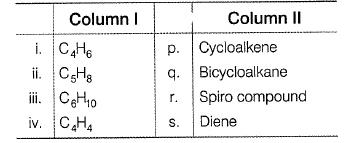Codes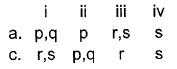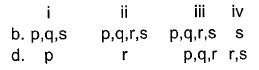Solution:
*Answer can only contain numeric values
QUESTION: 15

Direction (Q. Nos. 15-18) This section contains 4 questions. when worked out will result in an integer from 0 to 9 (both inclusive)

Q.

How manyisomers, each contaning a phenyl ring, are possible for C9H12 ?

Solution:

Double bond equivalent = 20-12/4 = 4
For phenyl ring, Double bond equivalent = 4(3 double bond and 1 ring)
So, we won’t have any double bond outside the ring.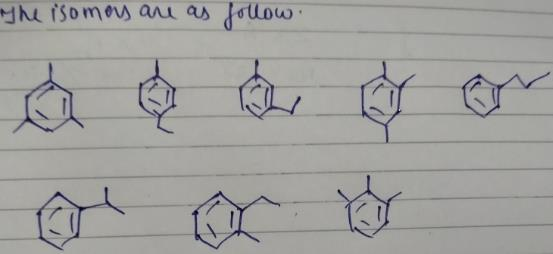*Answer can only contain numeric values
QUESTION: 16

How many positional isomers exist for C3H5Cl3 ?

Solution:

Explanation:

1,1,1-trichloropropane;

1,2,3-trichloropropane;

1,2,2-trichloropropane;

1,1,2-trichloropropane; this has 2 stereoisomers, with the chiral centre at C2;

1,1,3-trichloropropane;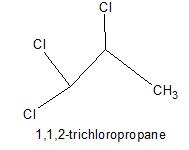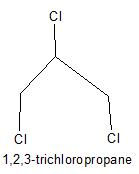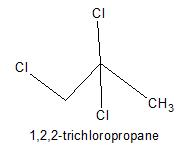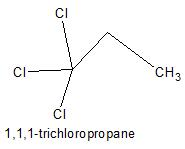*Answer can only contain numeric values
QUESTION: 17

How many enols (mono plus dienols only) excluding stereomers are possible for heptane-2,4-dione?

Solution:
*Answer can only contain numeric values
QUESTION: 18

How many ester isomers are possible for compound with molecular formula C5H10O2

Solution: